Next: Energy and momentum in SRT Up: Notions of relativistic dynamics Previous: The notion of the center of masses   Contents

## Forces in SRT

SRT gives nothing useful in the kinematics and for dynamical concepts as well. It occurs that all this huge number of additional complications arises only because of the fact, that the electromagnetic Lorentz force too "complicatedly" depends on the velocity (and on acceleration as well, if we will try to reduce its effect to the classical Newtonian second law)?! We will make a light lyrical digression. On what values can forces be dependent (and, from a conceptual point of view, in what a matter does the distinction of Newton's approach from the Aristotelian one lie)? An interaction of bodies leads to a change of the bodies' state. It is necessary to choose an "indicator" of this change. Aristotel believed the rest as the basis state, and he chose to observe the velocity of body's motion, as an indicator, i.e.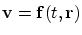(Aristotel connected the value of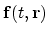with a force, provoking motion). The choiceis quite sufficient, if we will be satisfied with contemplation. However, if we would try to construct the dynamics of motion, so, after mental Galilean experiment, it became clear that the Aristotelian concept of the force was not conformed to the Reality. Though, strictly speaking, this conclusion is tied to the faith of relativists of "the first wave" - Galilean followers - in existence of empty space (Galilei by itself considered isolated identical systems only and, by contrast to his "pseudo-followers", he not disseminated his principle to mutually penetrating reference systems). If ether exists, the Aristotelian rest is locally tied to the ether, which has no necessity to be "uniformly immovable" as a whole at all, but can participate in complex vortical movements. For example, there exists the theory of vortical dynamics of the solar systems, and a force is only required to maintain motion, which is differ from equilibrium one. However, the analysis of vortical dynamics is not included in the book plan, and so, we will use the statements generally accepted in the present state. The Newtonian choice for the description of bodies' interaction is different - an "indicator" of change of body's state is chosen its acceleration. Factually, the Newton second law presents a definition of the notion of "a force", and, from a standpoint of functional dependence, the force coincides with the acceleration within a dimensional factor (mass). Ideally, this way of a motion description (in the habitual form) must be written as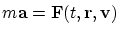. However, the problem of finding the explicit expression for such the "ideal" forces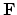is not yet solved for the case of arbitrary configurations and motions of a body, a force source and a medium, for example, based on expressions for statical forces. Nature does not easily unravel its secrets for us: instead of the ideal expression for the force, we are obliged to use an expression that we found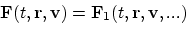. Thus, generally speaking, the real forces should be determined from the experiment. The following forces are known: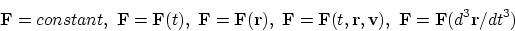and so on in quite various combinations. From the generalized expression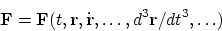it is seen that any derivative, including the second one, is not distinguished by anything, and only the experiment can determine the varieties of forces realized in the nature (recall, for example, the formula with an acceleration dependent force offered by Weber long before SRT). Here we are interested in the fact that the relativistic equation of motion with the Lorentz force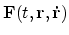can be written as the classical second Newton's law with the force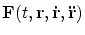. Though, if one believes in the relativistic expression for forces, then, as an alternative, it can be introduced transformations for components of the force, longitudinal and perpendicular to body's velocity (but it does not worth to introduce mythical longitudinal and transverse masses); or we can just write the classical second Newton law F=ma and the relationship of new force F and the static force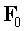: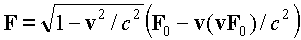. One should not also exaggerate the possibilities of the methods for obtaining expressions from the Lagrangian, since this function itself is determined to an accuracy of some expansion terms and can not determinate the principles.

Completely unclear methodically looks in SRT the transformation of forces at transition from one frame of reference to another. Let us consider, for example, two identical in absolute value charges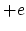and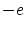being at distance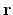apart of each other (Fig. 4.2).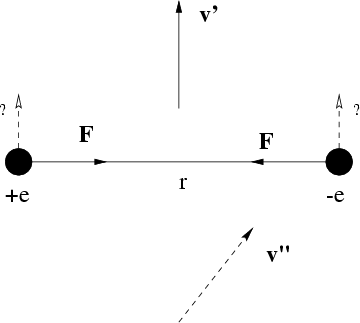In the frame of reference bound with resting charges there exists the electric force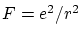acting between the charges. Look now at the same charges from the system moving at velocity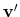perpendicular to the line connecting the charges (in this system the charges are flying parallel to each other). According to SRT [17,32], now between the charges acts the force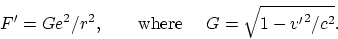To what physical quantity should be related the transformation factor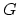? The charge is invariant in SRT. Distance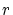, which is perpendicular to the motion, does not change as well. So, do the forces really lose their physical causes in SRT? Note one more strange thing: if the velocity of a observer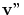has a component along the line which connects the charges, the force acting on the charges has a component which is perpendicular to this line (i.e. the picture of motion is essentially changed).

Completely groundless is Einstein's statement, that uncharged bodies must behave under an effect of forces in exactly the same manner as charged ones: all forces must be transformed identically. Still Poincare wrote that we can not arbitrarily "disconnect" some force from one body and arbitrarily "connect" it to the other body. If some force (for example, electrical) acts on some (charged) bodies and does not act at all on the other (uncharged) bodies, then, all the more, is not obvious that velocity dependencies should be identical in transformations of all forces. This is one more hypothesis not confirmed by anything even within the SRT framework. Probably, the transformation of forces has relation to only one particular case - the Lorentz force. And even in this case there are some nuances here. For example, at transition to a moving system the magnetic force magnitude can become zero. These facts represent the manifestations of conventional character of separating a single force into electrical and magnetic forces, don't they? In such a case, why the attention should be concentrated on the transformation of conventionally separated electrical and magnetic fields (and forces)?

Generally speaking, the idea that the same force can be different for different systems of observation is the flat nonsense for all experimental physics. Really, the way of writing arabic cipher on a dynamometer is independent on observer motion, i.e. readings of the dynamometer (fixing the force) will not be changed with observer motions. Any force acts between the "source" of this force and the concrete "object" of the applied force, and motion of some "strange eyes" has no relation at all (i.e. force can depends on the source properties, object properties, and their mutual motion).Next: Energy and momentum in SRT Up: Notions of relativistic dynamics Previous: The notion of the center of masses   Contents
Sergey N. Arteha